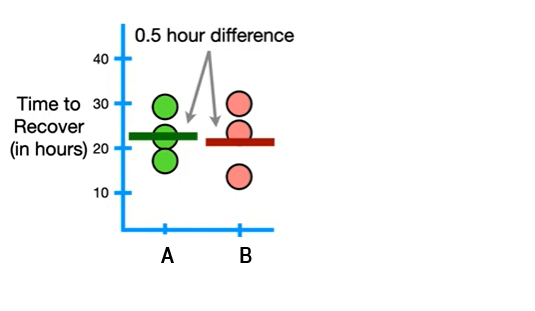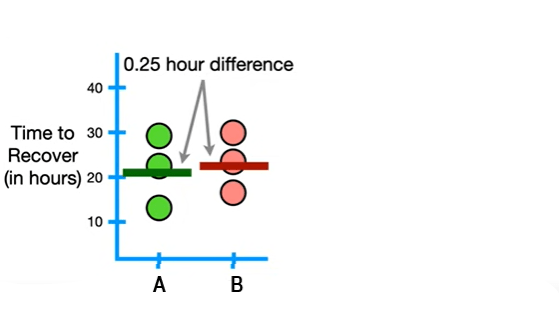×

Search anything:

# Different types of Hypotheses

#### data scienceIndian Technical Authorship Contest starts on 1st July 2023. Stay tuned.

• Introduction
• Null hypothesis
• Alternative hypothesis
• Simple hypothesis
• Complex hypothesis
• Directional hypothesis
• Non-directional hypothesis

# Introduction

A hypothesis is a precise, testable statement of what a researcher predicts will be the outcome of an experiment or study. The formulated hypothesis can further be tested to see whether it holds good or not. This usually involves proposing a relationship between the dependent (what the study measures or its outcome) and independent variables (the variables that are adjusted in the course of the study).

The main idea behind developing a hypothesis is that it helps us assume the probability of success and failure in the study and gives us a direction for the next steps. Apart from this, it helps us measure the validity of our study and provides us with the basic evidence to check the same.

There are six types of hypotheses that are widely used. They are null hypothesis, alternative hypothesis, simple hypothesis, complex hypothesis, directional hypothesis and non-directional hypothesis.

# Null hypothesis

The hypothesis that there is no difference between things is called the null hypothesis.

Suppose we are testing two drugs A and B for the same disease. We give the first drug A to 3 people and B to another 3 people suffering from the same disease and check the time required for them to recover from the disease. The null hypothesis is that there is no difference in recovery times between drug A and drug B. Let us visualize our results.We find that there is a 0.5 hour difference between the mean recovery times of drugs and B. Now, suppose we repeat the experiment with a new set of 6 people.This time, we find that the difference in mean recovery times of both the drugs is 0.25 hour. We know that the recovery time of a person is influenced by various factors that we cannot control like their diet, physical fitness, stress factor, state of their immune system and many more. Here, we can use the null hypothesis so we don't have to worry about whether the time difference is 0.25 hour or 0.5 hour. Instead, we simply see if the data convinces us to rehect the hypothesis that there is no difference between Drugs A and B.

Since small random factors affect the data, we fail to reject the null hypothesis in this case. But if the data is not affected by other small factors and that there is a large difference, then in that case we reject the null hypothesis. This is usually done by performing statistical tests.

# Alternative hypothesis

Alternative hypothesis states that there is a relationship present between the independent and dependent variables and that one variable has an effect on the another. It also states that the results are not due to chance and that they are significant in terms of supporting the theory that is being investigated. In layman's terms, alternative hypothesis are usually the opposite of null hypothesis.

So, in our above case of drug experimentation, the alternate hypothesis would be that there is a difference between the recovery times of drugs A and B.

When we have three or more groups, the concept of alternative hypothesis becomes more interesting. Suppose, instead of just two different drugs, we use 3 different drugs A,B and C in our previous study. The null hypothesis would be that there is no difference between all the 3 drugs. But the alternate hypothesis could take different forms! It can be that all the three drugs are different from each other, or that any two of the drugs are same and the third one is different.

# Simple hypothesis

A hypothesis in which all there exists a relationship between the dependent and independent variables , the form of probability distribution and the parameters involved (if any) are specified is known as simple hypothesis. It is important to note that the relationship is specified only between two variables here - one independent and one dependent.

"Smoking leads to cancer" is an example of simple hypothesis.

# Complex hypothesis

This is also known as composite hypothesis. This hypothesis states the relationship between two or more variables and does not specify the population completely - the form of probability distribution and some parameters remains unknown.

"Smoking and other drugs lead to cancer, chest infections, problems in throat, mental stress, etc" is an example of complex hypothesis.

# Directional hypothesis

A one-tailed directional hypothesis predicts that the independent variable affects the dependent variable and also predicts the nature or direction of the effect that the independent variable will have on the dependent variable.

For example, the experimenter hypothesizes that the adult participants would be able to recall more numbers correctly than the children in a memory game.

# Non-directional hypothesis

A two-tailed non-directional hypothesis predicts that the independent variable affects the dependent variable, but the direction of the effect it how it affects is not specified.

For example, the experimenter may hypothesize that there will be a difference in how many numbers are being correctly recalled by adults and children. He does not predict which one of them will perform better but just states that there will be a difference in their performances.#### Sanjana Babu

Sanjana Babu is a Student at SRM Valliammai Engineering College and is an Intern at OpenGenus. She is a Philomath.